# Texas Go Math Grade 2 Lesson 1.4 Answer Key Model 3-Digit Numbers

Refer to our Texas Go Math Grade 2 Answer Key Pdf to score good marks in the exams. Test yourself by practicing the problems from Texas Go Math Grade 2 Lesson 1.4 Answer Key Model 3-Digit Numbers.

## Texas Go Math Grade 2 Lesson 1.4 Answer Key Model 3-Digit Numbers

Explore

Use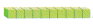. Draw to show what you did.

FOR THE TEACHER • Read the following problem. Jack has 12 tens blocks. How many hundreds and tens do Jack have? Have children show Jacks blocks and then draw quick pictures. Then have children circle 10 tens and solve the problem.
12*10=120
1 is in the hundreds place
2 is in the tens place
0 is in one’s place.
There will be 1 hundred blocks, 2 tens blocks, and 0 ones.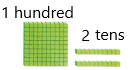Math Talk
Mathematical Processes

If Jock had 14 tens. how many hundreds and tens would he have? Explain
Answer: 14 tens is nothing but 140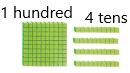14*10=140
1 is in the hundreds place
4 is in the tens place
0 is in one’s place.
There will be 1 hundred blocks, 4 tens blocks, and 0 ones.

Model and Draw

In the number 348, the 3 is in the hundreds place, the 4 is in the tens place, and the 8 is in the ones place.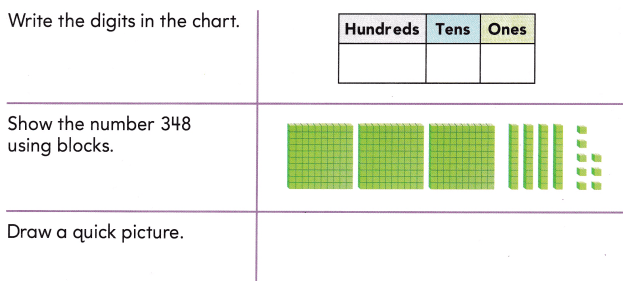Share and Show

Write how many hundreds, tens, and ones. Show with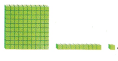. Then draw a quick picture.

Question 1.
234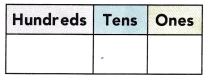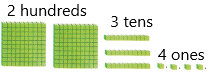There are 2 blocks of hundreds which means each such block is equivalent to 100
There are 3 blocks of tens which means each such block is equivalent to 10
There are 4 blocks of one’s which means each such block is equivalent to 1.
Place value: In Mathematics, place value charts help us to make sure that the digits are in the correct places. To identify the positional values of numbers accurately, first, write the digits in the place value chart and then write the numbers in the usual and the standard form.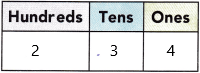The value of digits of 234:
2 is in the hundreds of place
3 is in tens place
4 is in one’s place

Question 2.
156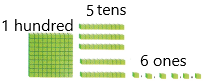There are 1 block of hundreds which means each such block is equivalent to 100
There are 5 blocks of tens which means each such block is equivalent to 10
There are 6 blocks of one’s which means each such block is equivalent to 1.
Place value: In Mathematics, place value charts help us to make sure that the digits are in the correct places. To identify the positional values of numbers accurately, first, write the digits in the place value chart and then write the numbers in the usual and the standard form.The value of digits of 156:
1 is in the hundreds place
5 is in the tens place
6 is in one’s place.

Problem Solving

Write how many hundreds, tens, and ones. Show with. Then draw a quick picture.

Question 3.
125There are 1 block of hundreds which means each such block is equivalent to 100
There are 2 blocks of tens which means each such block is equivalent to 10
There are 5 blocks of one’s which means each such block is equivalent to 1.
Place value: In Mathematics, place value charts help us to make sure that the digits are in the correct places. To identify the positional values of numbers accurately, first, write the digits in the place value chart and then write the numbers in the usual and the standard form.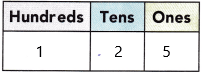The value of digits of 125:
1 is in the hundreds place
2 is in the tens place
5 is in one’s place.

Question 4.
312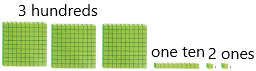There are 3 blocks of hundreds which means each such block is equivalent to 100
There are 1 block of tens which means each such block is equivalent to 10
There are 2 blocks of one’s which means each such block is equivalent to 1.
Place value: In Mathematics, place value charts help us to make sure that the digits are in the correct places. To identify the positional values of numbers accurately, first, write the digits in the place value chart and then write the numbers in the usual and the standard form.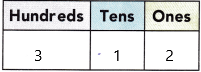The value of digits of 312:
3 is in the hundreds place
1 is in tens place
2 in one’s place.

Solve.

Question 5.
H.O.T. How are the numbers 342 and 324 alike? How are they different?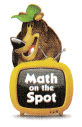Place value: In Mathematics, place value charts help us to make sure that the digits are in the correct places. To identify the positional values of numbers accurately, first, write the digits in the place value chart and then write the numbers in the usual and the standard form.342: 3 is in the hundreds place
: 4 is in the tens place
: 2 is in one’s place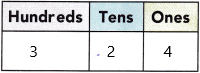324: 3 is in the hundreds place
: 2 is in the tens place
: 4 is in one’s place

Question 6.
H.O.T. Multi-Step I have 10 hundred blocks, 5 one’s blocks, and 8 tens blocks. I use all my blocks to model two 3-digit numbers. What could my two numbers be?
__________ and ___________
There are 10 blocks of hundreds which means each such block is equivalent to 100
There are 5 blocks of tens which means each such block is equivalent to 10
There are 8 blocks of ones which means each such block is equivalent to 1.
The total of these blocks will be:10(100)+5(10)+8(1)
The total blocks are 1058.

Question 7.
Analyze Mr Martin’s cookie order includes 6 boxes of 100 cookies. Which could be the number of cookies in his order?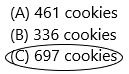Explanation:
The number of boxes of cookies he includes in order=6
The number of cookies in each box=100
Here asked the question this 600 cookies also included and in tens and ones place might have some number of cookies. Here 600 cookies are given so I prefer option c. And moreover, 6 is in the hundreds place.

Question 8.
Connect Elmo delivers 7 boxes of 10 cookies, 3 boxes of 100 cookies, and 4 single cookies. How many cookies does Elmo deliver?(A) 347
(B) 734
(C) 374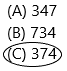Explanation:
There are 3 boxes of hundred cookies which mean each such box is equivalent to 100
There are 7 boxes of ten cookies which means each such box is equivalent to 10
There are 4 boxes of single cookies which mean each such block is equivalent to 1.
The total of these blocks will be:3(100)+7(10)+4(1)
The total blocks are 374

Question 9.
TEXAS Test Prep What number is shown with these blocks?(A) 342
(B) 243
(C) 432Explanation: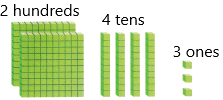There are 2 blocks of hundreds which means each such block is equivalent to 100
There are 4 blocks of tens which means each such block is equivalent to 10
There are 3 blocks of one’s which means each such block is equivalent to 1.
The total of these blocks will be:2(100)+4(10)+3(1)
The total blocks are 243

TAKE-HOME ACTIVITY • Write the number 438. Have your child tell you the values of the digits in the number 438.Place value: In Mathematics, place value charts help us to make sure that the digits are in the correct places. To identify the positional values of numbers accurately, first, write the digits in the place value chart and then write the numbers in the usual and the standard form.
438: 4 is in the hundreds place
: 3 is in the tens place
: 8 is in one’s place

### Texas Go Math Grade 2 Lesson 1.4 Homework and Practice Answer Key

Write how many hundreds, tens, and ones. Show with. Then draw a quick picture.

Question 1.
242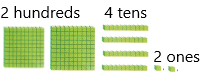Explanation:
There are 2 blocks of hundreds which means each such block is equivalent to 100
There are 4 blocks of tens which means each such block is equivalent to 10
There are 2 blocks of one’s which means each such block is equivalent to 1.
The total of these blocks will be:2(100)+4(10)+2(1)
The total blocks are 242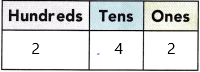Place value: In Mathematics, place value charts help us to make sure that the digits are in the correct places. To identify the positional values of numbers accurately, first, write the digits in the place value chart and then write the numbers in the usual and the standard form.
242: 2 is in the hundreds place
: 4 is in the tens place
: 2 is in one’s place

Question 2.
483There are 4 blocks of hundreds which means each such block is equivalent to 100
There are 8 blocks of tens which means each such block is equivalent to 10
There are 3 blocks of one’s which means each such block is equivalent to 1.
Place value: In Mathematics, place value charts help us to make sure that the digits are in the correct places. To identify the positional values of numbers accurately, first, write the digits in the place value chart and then write the numbers in the usual and the standard form.
483: 4 is in the hundreds place
: 8 is in the tens place
: 3 is in one’s place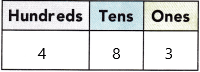Problem Solving

Question 3.
How are the numbers 579 and 597 different?
Explanation: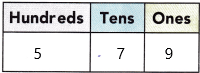Place value: In Mathematics, place value charts help us to make sure that the digits are in the correct places. To identify the positional values of numbers accurately, first, write the digits in the place value chart and then write the numbers in the usual and the standard form.
579: 5 is in the hundreds place
: 7 is in the tens place
: 9 is in one’s place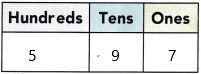597: 5 is in the hundreds place
: 9 is in the tens place
: 7 is in one’s place
Therefore, the place value makes the difference in both numbers.

Question 4.
Multi-Step I have 7 hundred blocks, 5 tens blocks, and 8 one’s blocks. I use my blocks to model two 3-digit numbers. What could my two numbers be?
_________ and ___________
Explanation:
There are 7 blocks of hundreds which means each such block is equivalent to 100
There are 5 blocks of tens which means each such block is equivalent to 10
There are 8 blocks of ones which means each such block is equivalent to 1.
The total of these block will be:7(100)+5(10)+8(1)
The total blocks are 758.

Lesson Check

Question 5.
Lexi needs 144 beads. A large box holds 100 beads. A medium box holds 10 beads. A small box holds 1 bead. Lexi already has 1 large box and 4 small boxes. How many medium boxes of beads does she need?
(A) 104
(B) 4
(C) 40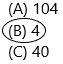Explanation:
The total number of needs Lexi needs=144
The number of beads a large box can hold=100
The number of beads a medium box can hold=10
The number of beads a small box can hold=1
The number of large boxes she has already=1
The number of small boxes she has already=4
The number of medium boxes she has=X
We need to find out the number of medium boxes so that he could have 144 beads.
As we know 1 large box=100 beads
1 small box=1 bead, likewise 4 small boxes means Lexi is having 4 beads.
The total number of beads he has now=100+4=104.
Now to calculate the remaining beads we need to subtract the total beads he needs and the beads he has now.
Now we got the remaining beads from this we can calculate the medium boxes.
X=40/10
X=4.
Therefore, she needs 4 medium bags.

Question 6.
Carla’s bead order includes 3 boxes of 100 beads. Which could be the number of beads in Carla’s order?
(A) 263
(B) 230
(C) 314Explanation:
The number of boxes of beads he includes in order=3
The number of cookies in each box=100
Here asked the question this 300 beads also included and in tens and ones place might have some number of cookies. Here 300 cookies are given so I prefer option c. And moreover, 3 is in the hundreds place.

Question 7.
Tess has 2 boxes of 10 beads, 4 boxes of 100 beads, and 6 boxes of single beads. How many beads does Tess have in all?
(A) 642
(B) 426
(C) 246Explanation:
The number of boxes of beads Tess have=2 (medium box)
The number of beads in each box=10
The total number of beads in 2 boxes=2*10=20
The number of boxes of beads Tess have=4
The number of beads in each box=100 (large boxes)
The total number of beads in 4 boxes=4*100=400
The number of boxes of beads Tess have=6 (small boxes)
The number of beads in each box=1
The total number of beads in 6 boxes=6*1=6
20+400+6
=426.

Question 8.
Dave made a drawing of 4 hundred blocks, 3 one’s blocks, and 7 tens blocks. What number did he model?
(A) 473
(B) 743
(C) 374